## #StackBounty: #code-challenge #sequence #geometry #polyomino Counting generalized polyominoes

### Bounty: 100

This challenge will have you count pseudo-polyforms on the snub square tiling.

I think that this sequence does not yet exist on the OEIS, so this challenge exists to compute as many terms as possible for this sequence.

# Definitions

The snub square tiling is a semiregular tiling of the plane that consists of equilateral triangles and squares.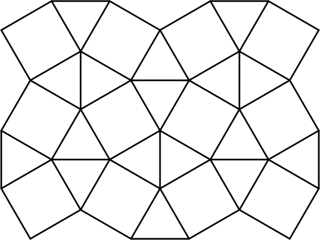A pseudo-polyform on the snub square tiling is a plane figure constructed by joining together these triangles and squares along their shared sides, analogous to a polyomino. Here is an example of a six-cell and an eight-cell pseudo-polyform:# Examples

For `n = 1` there are two 1-cell pseudo-polyforms, namely the square and the triangle: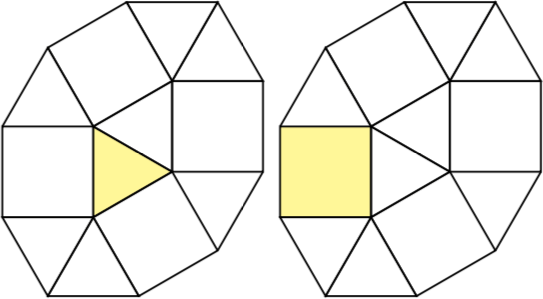For `n = 2` there are two 2-cell pseudo-polyforms, namely a square with a triangle and two triangles.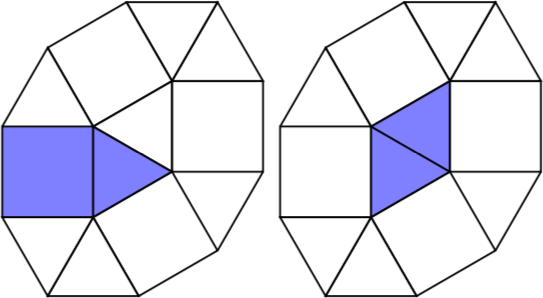For `n = 3` there are four 3-cell pseudo-polyforms.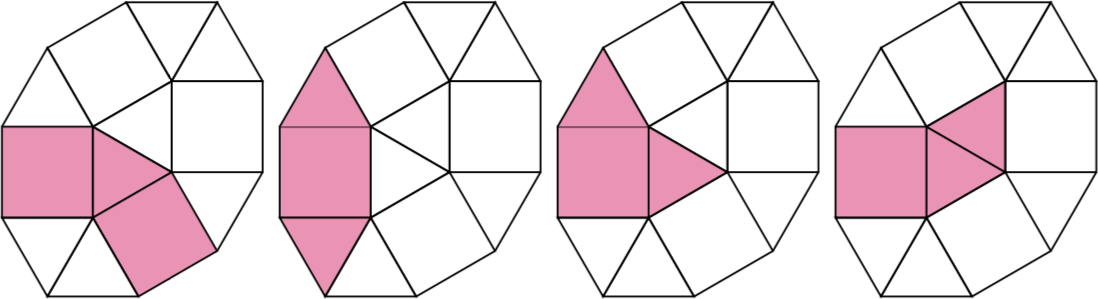# Challenge

The goal of this challenge is to compute as many terms as possible in this sequence, which begins `2, 2, 4, ...` and where the n-th term is the number of n-cell pseudo-polyforms up to rotation and reflection.

Run your code for as long as you’d like. The winner of this challenge will be the user who posts the most terms of the sequence, along with their code. If two users post the same number of terms, then whoever posts their last term earliest wins.

(Once there are enough known terms to prove that this sequence does not already exist in the OEIS, I’ll create an entry in the OEIS and list the contributor as a co-author if he or she desires.)

Get this bounty!!!

### Bounty: 250

Although you don’t need to know Haskell language to do this challenge, Haskellers might have an advantage here.

Point-free means roughly that variables(points) are not named and the function is created from compositions of others functions.

For example, in Haskell, the function `f : x->x+1` can be defined by:
`f x = x+1` which uses the variable `x` and is not point-free. But it can be simplified to its point-free version: `f=(+1)`. Likewise, you have `f x=x^k` -> `f=(^k)`, and `f x=x*k` -> `f=(*k)`, `f x y=x*y` -> `f=(*)`

More complex functions can be constructed using the Haskell’s function composition operator `(.)`:

`f x = x->2*x+1` is converted to `f = (+1).(*2)`: Multiply by 2 then add 1.

In this challenge you will have to create a point-free style Haskell code generator.
This generator will take a polynomial function of multiple variables as input and output corresponding code in Haskell language and in point-free style.

This is way harder than the previous simple functions, so if you don’t know Haskell at all, or if you are not comfortable with operators `(.)`, `(>>=)`, `(<*>)` on functions, You should follow the Construction Process which is detailled bellow or start directly from its Python Implementation.

## Example

Say you want to write the polynomial function `x, y -> x²+xy+1` point-free.

Your program might output the folowing code:

``````((<*>).(.)((<*>).(.)(+)))                           -- sum 2 functions of 2 variables
(
((<*>).(.)((<*>).(.)(+)))                   -- sum 2 functions of 2 variables
(
(
((.)((.(^0)).(*)))              -- g x y = y^0 * f x   => g x y = x^2
(((.(^2)).(*))(1))              -- f x = 1*x^2
)
)
(
((.)((.(^1)).(*)))                  -- g x y = y^1 * f x   => g x y = x*y
(((.(^1)).(*))(1))                  -- f x = 1*x^1
)
)
(
((.)((.(^0)).(*)))                          -- g x y = y^0 * f x   => g x y=1
(((.(^0)).(*))(1))                          -- f x = 1*x^0
)
``````

## Construction Process:

One possible algorithm is to first write each monomial function in point-free style (step 1), then create operators to add functions two at a time (step 2), and apply this to the list of monomials, writing `f+g+h` as `(f+(g+h))` (step 3).

1. Write each monomial, taking each of the factors successively. Example: `11*a^12*b^13*c^14`.
• Start with `(11)`, a 0-argument function.
• Take the previous function `f0` and write `((.(^12)).(*))(f0)`; this is a 1-argument function `a->11*a^12`
• Take the previous function `f1` and write `((.)((.(^13)).(*)))(f1)`; this is a 2-argument function `a->b->11*a^12*b^13`.
• Finally, take the previous function `f2` and write `((.)((.)((.(^14)).(*))))(f2)`. Thus `a->b->c->11*a^12*b^13*c^14` is converted to point-free style as `((.)((.)((.(^14)).(*))))(((.)((.(^13)).(*)))(((.(^12)).(*))(11)))`.
• In general, continue transforming `f` to something of the form `((.) ((.) ((.) ... ((.(^k)).(*)) ))) (f)` until your monomial is built and takes the desired number of arguments.
• Warning: If using this construction algorithm, each monomial must take the same number of arguments. Add some `^0` for missing variables (`a^2*c^3` -> `a^2*b^0*c^3`)
• You can test your monomial generator here with this example and the next iteration: Try it online!
2. Create the operator $$O_n$$ that adds two functions of $$n$$ arguments:
• $$O_0$$ is `(+)`
• For $$ngeq0$$, $$O_{n+1}$$ can be constructed recursively as `((<*>).(.)( O_n ))`
• E.g. `((<*>).(.)(((<*>).(.)(+))))` adds two 2-argument functions.
3. Chain the addition: The operator from step 2 only adds two monomials, so we must manually fold addition. For example, for monomials f, g, h, write `f+g+h` => `O_n(f)( O_n(g)( h ) )` replacing f, g, and h with their point-free equivalents from step 1, and $$O_n$$ by the correct operator from step 2 depending on the number of variables.

## Implementation in Python

This is an ungolfed implementation in Python 3 which reads polynomials as list of monomials where monomial is a list of integers:

``````def Monomial(nVar,monoList):
if nVar==0:
return "({})".format(monoList)
else:
left = "((.(^{})).(*))"
mono = "({})".format(monoList)
for i in range (0,nVar):
p = monoList[i+1] if i+1<len(monoList) else 0
mono = left.format(p) + "(" + mono + ")"
left = "((.)" + left + ")"
return mono

def Plus(nVar):
if nVar==0:
return "(+)"
else:
return "((<*>).(.)(" + Plus(nVar-1) + "))"

def PointFree(l):
nVar = 0
for monoList in l:
if nVar<len(monoList)-1: nVar = len(monoList)-1

pointfree = Monomial(nVar,l)

plusTxt = Plus(nVar)

for monoList in l[1:]:
pointfree = plusTxt + "(" + Monomial(nVar,monoList) + ")(" + pointfree + ")"

return pointfree
``````

## Input

The input is the polynomial function to be converted.

The input format shall consist of a list of Integers in any format you want.
For example, you might define the polynomial `5*a^5+2*a*b^3+8` as the list `[[5,5,0],[2,1,3],]`

## Output code

The output is the Haskell code for that polynomial as a function of n Integers,

written in point-free style with only numeric literals and base functions(no Import).

Of course, the above process and implementation comply to these rules.

For Haskell programmers, this means no list comprehension, no lambdas and only Prelude functions(no import, language extensions..):
`(+) (-) (*) (^) (.) (<*>) (>>=) flip sum zip uncurry curry (\$) ...`

## Test cases

Your program should work for all valid inputs, but will be scored on on these five polynomials:

``````f1 a b c d = 5*a^4*b^3*c^2 + a^2*b^3*c^4*d^5
f2 a b c   = -5*a^5*c^8 + 3*b^3*c^4 + 8
f3 a b     = 5*a^5+3*b^3+8
f4 a       = a^3+a^2+a+1
f5         = 1+2-3
``````

Tests cases with the Python code given above:Try it online!

Once you have run your program on these samples, copy/paste your output code into the following program (in the “Code” section) and run it in order to check your outputs:

Try it online!

If your functions are correct, it will output:

``````f1 : +++ OK, passed 100 tests.
f2 : +++ OK, passed 100 tests.
f3 : +++ OK, passed 100 tests.
f4 : +++ OK, passed 100 tests.
f5 : +++ OK, passed 100 tests.
``````

## Scoring

Code length + (sum of the test cases output length)/8.

-300 if your program consist in a point-free style Haskell function (with the same rules as for output).

I’ll give a Bounty of 250 points if you beat my score of 73.125 (272 + 809/8 – 300).

The Python solution given above is 797 bytes long, and outputs functions of length: 245, 282, 180, 132, and 24 so the score would be `797 + (245+282+180+132+24)/8 = 904.875`.

The smallest score wins!!

Get this bounty!!!

# Background

The traveling salesman problem (TSP) asks for the shortest circuit that visits a given collection of cities. For the purposes of this question, the cities will be points in the plane and the distances between them will be the usual Euclidean distances (rounded to the nearest integer). The circuit must be “round-trip”, meaning it must return to the starting city.

The Concorde TSP solver can solve instances of the Euclidean traveling salesman problem, exactly and much faster than one would expect. For example, Concorde was able to solve an 85,900-point instance exactly, parts of which look like this: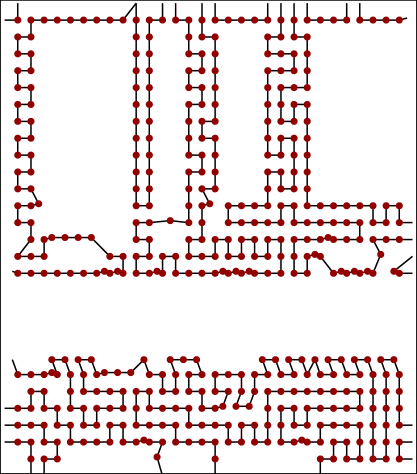However, some TSP instances take too long, even for Concorde. For example, no one has been able to solve this 100,000-point instance based on the Mona Lisa. (There is a \$1,000 prize offered if you can solve it!)

Concorde is available for download as source code or an executable. By default, it uses the built-in linear program (LP) solver QSopt, but it can also use better LP solvers like CPLEX.

# The challenge

What is the smallest TSP instance you can generate that takes Concorde more than five minutes to solve?

You can write a program to output the instance, or use any other method you would like.

# Scoring

The fewer points in the instance the better. Ties will be broken by the file size of the instance (see below).

# Standardization

Different computers run faster or slower, so we will use the NEOS Server for Concorde as the standard of measurement for runtime. You can submit a list of points in the following simple 2-d coordinate form:

``````#cities
x_0 y_0
x_1 y_1
.
.
.
x_n-1 y_n-1
``````

The settings that should be used on NEOS are “Concorde data(xy-list file, L2 norm)”, “Algorithm: Concorde(QSopt)”, and “Random seed: fixed”.

# Baseline

The 1,889-point instance `rl1889.tsp` from TSPLIB takes “Total Running Time: 871.18 (seconds)”, which is more than five minutes. It looks like this:

Get this bounty!!!